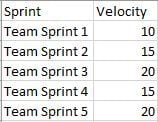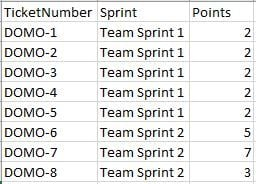# Calculating on a calculation

Couldn't think of the best way to title this but the description should make it clear. The calculation I need to do is a basic one but doing it isn't as straight forward as it seems.

I'd like to calculate an average of the total work a team gets done in a set period of time and if you're familiar with the software dev world I'm calculating the velocity (work done) per sprint (2 week cycle of work). I can calculate it on a per sprint number just fine however I'm trying to calculate the average velocity for a quarter but the catch is I can't just use the same calculation because it will just do the avg of all rows divided by the number of sprint. I want an average of the previous averages I calculated.

Ex. Here is data I already have calculated where velocity is a SUM of certain individual work items that are part of the same sprint, Ex. 5 tickets worth 2 points each were in sprint 1 so the velocity a 10 is a SUM of all the points for sprint 1.My goal is to have an average velocity per quarter which I want to be 10+15+20+15+20/5 = 16

What I don't want it to do (which it normally would given my underlying data) is to SUM all the individual tickets for all sprints (2+2+2+2+2+5+1+4+6...etc) / 5

Example underlying data structure where you can see for the above Team Sprint 1 = 10 that is a very simple SUM(`Points`) of all tickets by Sprint name.**Make sure to like any users posts that helped you and accept the ones who solved your issue.**

• Greetings,

few questions to get started:

Is your Velocity calculated via a beastmode?

Would you be calculating the Quarterly Average based on the current quarter we're in or are you wanting to see Averages by Quarter?

I'm assuming you have some kind of date field as to when the work was completed whcih then determines your quarter?

• The Velocity is calculated via a beastmode. It's basically a SUM of all the tickets that meet a certain criteria. I can include it if you'd find it useful but it's a little more detailed than the example I gave because I was trying to make it as simple to understand.

I'd be seeing averages by quarter so a Q1 number, Q2, Q3, Q4.

And yes I am using the end date of the sprint to know when things are in each quarter.

**Make sure to like any users posts that helped you and accept the ones who solved your issue.**
• Understood. testing something now, be back in a few ?

• using MySql I was able to get to your test average of 16 for Q1 (assuming all end dates were Q1 2019). Only downside is you lose the ability to drill down to the raw data through the card.

Thoughts?

• For drill down you can use a separate dataset that has the detail level data (and not the aggregated data). The only thing to keep in mind would be to have the same field in the detail dataset on which the user is going to drill on.

• I'll experiment with doing it as a dataflow and swapping datasets. Was wondering if I could work any beastmode magic to avoid it but yea it seems MySQL dataflow will be what I do. I'll update once I have something if I have an additional question

**Make sure to like any users posts that helped you and accept the ones who solved your issue.**